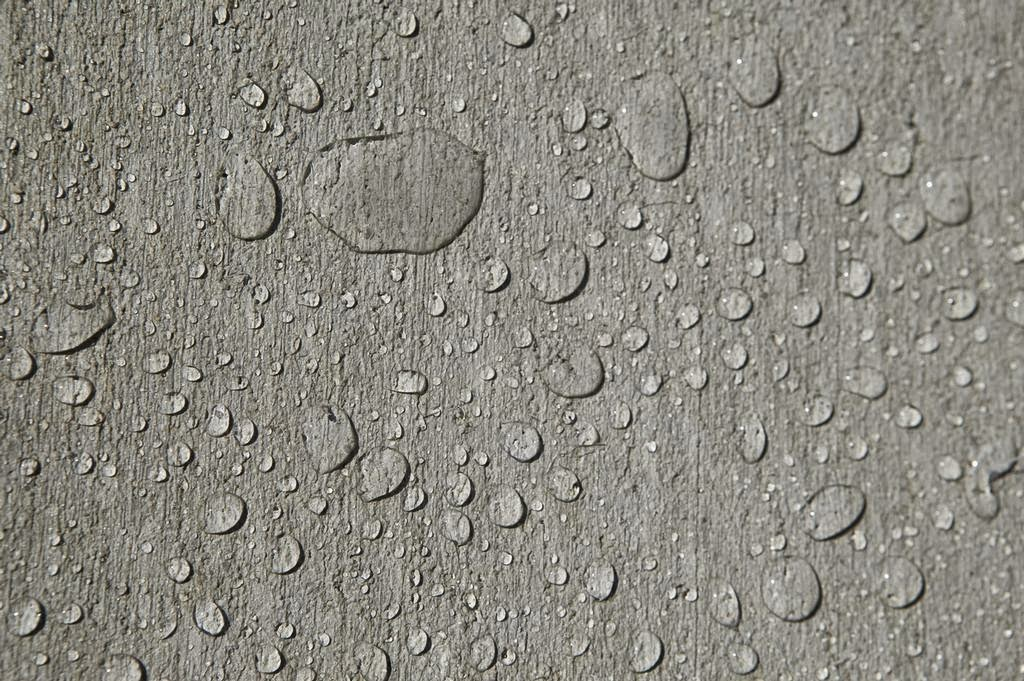## Recent Posts

The limit of water absorption by coarse aggregates is determined so as to calculate the appropriate amount of water to be added to the concrete mix, thus modifying the water cement ratio. A bone dry aggregate would necessitate, while for wet aggregate water contents should be reduced.
"Determine the water absorption by coarse aggregate "

## Designation of Experiment

BS-812, Part 2: 1975

## Apparatus

Balance, Oven, Dry and Soft absorbent cloth, shallow TraysDetermine the Water absorption of coarse aggregate

## Materials Required

Sample of watered aggregates and water.

## Procedure

Take a sample of coarse aggregates, wash to remove finer particles and dust and then immerse in water for 24+1/2 hours.
After this period, aggregate are taken out and dried with a dry absorbent cloth.
Weigh the aggregates and let this weight be A.
Place the aggregate in the oven at a temperature 100-110 for 24+1/2 Hours.
Remove the sample of aggregates from oven and cool in air, weight them and let this weight be B.

## Observations and Calculations

Percentage water absorption =( (A-B)/B ) x 100
Wehere
A = Mass of saturated surface dry sample
B = Mass of Oven dried sample
A = 2225 gms
B = 2170 gms
Percentage Water Absorption = (55/2225) x 100 =2.47 %
Result
Percentage water absorption of coarse aggregate of given sample is 2.472 %.